﻿ Impossible Escape?# Impossible Escape?

The other day I heard a really interesting puzzle.

It's one of those proverbial prisoner problems where you are condemned to die unless you can prove your intelligence to a devious jailer. You, and your friend, are incarcerated. Your jailer offers a challenge. If you complete the challenge you are both free to go. Here are the rules: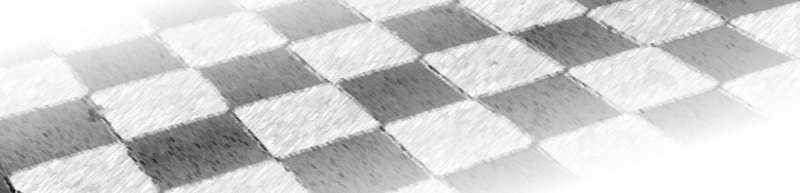• The jailer will take you into a private cell. In the cell will be a chessboard and a jar containing 64 coins.

• The jailer will take the coins, one-by-one, and place a coin on each square on the board. He will place the coins randomly on the board. Some coins will be heads, and some tails (or maybe they will be all heads, or all tails; you have no idea. It's all at the jailers whim. He may elect to look and choose to make a pattern himself, he may toss them placing them the way they land, he might look at them as he places them, he might not …). If you attempt to interfere with the placing of the coins, it is instant death for you. If you attempt to coerce, suggest, or persuade the jailer in any way, instant death. All you can do it watch.

• Once all the coins have been laid out, the jailer will point to one of the squares on the board and say: “This one!” He is indicating the magic square. This square is the key to your freedom.

• The jailer will then allow you to turn over one coin on the board. Just one. A single coin, but it can be any coin, you have full choice. If the coin you select is a head, it will flip to a tail. If it is a tail it will flip to a head. This is the only change you are allowed to make to the jailers initial layout.

• You will then be lead out of the room. If you attempt to leave other messages behind, or clues for your friend … yes, you guessed it, instant death!

• The jailer will then bring your friend into the room.

• Your friend will look at the board (no touching allowed), then examine the board of coins and decide which location he thinks is the magic square.

• He gets one chance only (no feedback). Based on the configuration of the coins he will point to one square and say: “This one!”

• If he guesses correctly, you are both pardoned, and instantly set free. If he guesses incorrectly, you are both executed.

• The jailer explains all these rules, to both you and your friend, beforehand and then gives you time to confer with each other to devise a strategy for which coin to flip.

What is your strategy. How do you escape?

### Is it possible to devise a strategy?

At first glance this problem seems impossible to solve. We have no control over the jailer. The board could have any layout before we get chance to flip our coin. To compound matters, we have no idea which square the jailer will point to. (In fact, the jailer himself probably does not know which square he is ultimately going to select as he is initially laying out the coins).

There a 64 possible squares he could point to. This is 26 possible answers. We need six binary pieces of information to distinctly identify the target square. If we flip a coin, this is one bit of information, enough to select between two choices (and this assumes we can even get this bit!) Because we can't transmit the before state of the board, there is no way for our friend to tell which coin is the one that has been turned over. Think about it, if your friend enters the room and sees 63 heads and 1 tail, there is no way for him to know if the solitary tail was the coin you flipped, or if when you encountered the board there were 62 heads and 2 tails and you flipped one of the tails over!

 Is it possible to communicate six bits of information by the flip of a single coin?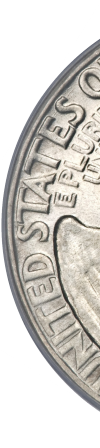There is a strategy that allows you to escape with 100% certainty irrespective of the state of the starting board and whatever magic square the jailer chooses. I encourage you to think about it for a moment even if you are going to read the answer without trying to solve

(Before you ask, the solution does not involve any kind of cheat, gotcha, trap or trick. It's a purely mathematical solution).### Start Small

It is possible to solve the problem because, in fact, there is more information than just one-bit being transmitted between you and your friend. The state of the other coins on the board is storing information, and we can exploit this. There are indeed sufficient controllable bits of information (six bits) in the coin layout we can use to provide just sufficient information to identify any square on the board.

To understand this we'll need some understanding of binary, exclusive or functions, and parity. I'll get into these shortly, but it's worth thinking about a smaller version of this problem first.

### Two Squares

Imagine we had a chessboard with just two squares. There are four possible ways the jailer could arrange the coins: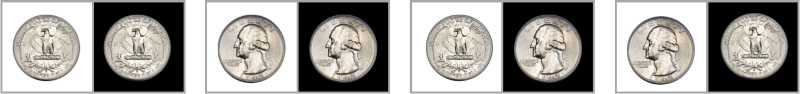These are TT, HH, TH and HT. The jailer can also make the magic square either the first square or the second square.

Beforehand, we agree on a rule. Our rule is that, if the jailer points to the first square (the white one), then I will make sure that a head is in the first square. Here are all the possible scenarios, and the coin I would flip:If the layout was TT, I could flip the first coin to make it a head. If it was HH, I'd flip the second coin, as the first was already a head (it makes no difference to our rule, but I have to flip a coin). If the initial board was TH, again I flip the first coin, and finally if HT, I flip the second coin.

In all the above, there is a head in the first square. Our rule has told us the jailer picked the first square.

Similarly, if the jailer points to the second square then I'll make sure the coin on the first square is NOT a head. This is the clue that tells my compatriot that the magic square is not the first one.In all the above, there is no head in the first square. Our rule has told us the jailer picked the second square.

Problem solved!

### Induction

Mathematicians (and some computer programmers), will tell you that this all we need to do to prove that a solution to the chessboard problem is possible. If we can encode one-bit of information (which of the two states the jailer chose) using two squares, then by induction we can show that it's possible to encode two-bits using four squares, or three-bits using eight squares … or six-bits using sixty-four squares. It's sort of like recursion in reverse: Starting with a small building block, we can nest these to make the next power of two.

1, 2, 4, 8, 16, 32, 64

Let's see if we see what this looks like.

### Binary

Because we are nesting based on powers of two, we can use binary to help represent/visualize the state of the board, and what combination of bits we need to look at.

 Here on the left is a depiction of a chess board. If we label the grid starting with zero in the top left, and sixty-three in the lower right then, using binary, we can represent which nested set each square is part of. The board on the left is interactive. Clicking on the grid shows the binary representation of that square. The number in the lower left shows the square in binary notation. Each digit represents the set that this square is in. A digit 1 in any position shows that square is in one half of a set, a digit 0 in the other half. By definition each square is unique and has a distinct collection of bits. ← click here We'll use this information later.

### Parity

Instead of using two squares, like in our simple example, we're dividing the chessboard into various combinations of two sets (like odd/even squares, based on the filters produced by the binary bit patterns). We need to apply our marking rule to these two regions/sets instead of to just one square. If the magic square is located in the first region we want to mark one way, and if it is present in the second region, we mark another way.

Since our regions are collections of squares, we can't simply make them all heads (we're only allowed to flip one coin). Instead we'll flip just one coin in a region. We can count the number of heads in the region we are interested in. If the total number of heads is odd, we'll define this as 1, if it is even we'll define this as zero. Because of the way numbers work, flipping any single coin in any region will change the number of heads in that region from odd to even, and vice versa (Adding, or subtracting one to any number inverts it's odd/even state).

This is the concept of parity, and toggling from one state to the other with the addition or subtraction of one bit is used by computers heavily. If there are an odd number of heads in a region we'll describe this as having a parity of one. If there are an even number, we'll describe this as having parity of zero.

To change the parity of a region, all we have to do is flip one coin in that region.

Using masks of powers of two, there are different ways to break a chessboard into two regions. Here are pictures of the various ways to divide the board based on the presence or not of that bit (power of two) of the square number. The superposition of these filters means it is possible to identify one square we can flip that will modify the parity of any/all of the bits.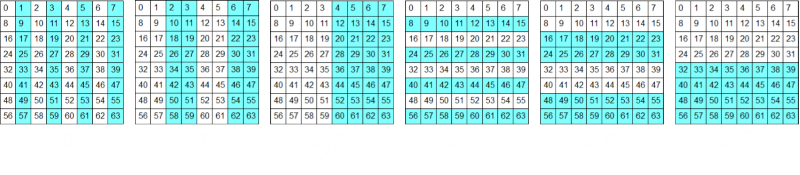20 is the same as logical AND with 000001, 21 is the same as logical AND with 000010, 22 is the same as logical AND with 000100 … 25 is the same as logical AND with 100000.

By definition, each square on the grid has has a unique bit pattern. It's possible to flip one coin to change the parity of any combination of these filters just by knowing which of these patterns the bits overlap. Are you starting to see a solution?The board that the jailer produces will have a natural parity. The coins are randomly placed, and we can calculate this parity pattern based on the six patterns/filters above, and the number of heads in each region. This combination of parity bits will depict a random six-bit number.

The magic square requires six-bits to describe, and we can encode this in the parity of the board.

We know that we can change any single coin and cause this to modify all/any the bits of the number. All we need to do is find the correct coin to flip over to change the combination of parity to depict the number we want to represent (the binary encoding of the magic square).

We're almost at our solution!

### Putting it all together

Let's put it all together with a little interactive model. Below are two depictions of the chessboard. Using the board on the left, you can click to select the magic square that the jailer selects. Below the board, in red, is the shown the target number of the board, and to the left of this is the representation of this in binary. Try it now!

The board on the right depicts the coins on the board. In the interests of clarity/contrast, I'm only showing the coins that are heads (the blank squares depict locations where there would be a coin showing tails). The board is showing random coins. You can manually click on the right grid to toggle any cell. To the right are two additional buttons. The "Random" button will re-fill the grid with a random collection of coins. The "Clear" button sets all the board to tails (This is useful to reset the board. This way you can add heads, one-by-one and see the effect on the board). Give it a try now.

Below the right grid, on the left, in gray, is the natural parity of the board. Finally, show in green is the calculated coin to flip. The green reticle on the board shows the location you need to flip to save your life! The green number underneath gives it's value, and this is also shown in binary.

### How are these values calculated?

We already know where the number on the left comes from. This is simply the binary description of the square. Each bit of the number represents if that coin is present, or not, in those power-of-two pattern filters we saw above.

On the right grid, the gray number is the natural parity of the board based on the coins present. For each of the power-of-two filters we find out if there is an odd, or even, number of heads in that region. A value is one in any bit location tells us there are an odd number of heads in that region.

The green number shows the square such that, if the state of this square were modified, the parity of the board would then show the desired target number. It's the single square that would change the bits from the random (known) value to the desired value. It is calculated by performing an XOR (eXclusive-OR) bitwise operation on the natural parity of the board and the desired value.

### XOR

The XOR (one or the other, but not both) operator is used heavily in computer programming. It has a couple of interesting properties.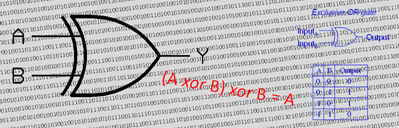If the XOR operator is applied twice with the same value it returns the input to its original state (A XOR B) XOR B = A Also, if you apply it against a number with all bits set, then it causes all the bits in the number to flip. XORing with a number that has a collection of bits set inverts those bits, and preserves the rest.

This is exactly why we use the XOR operator to determine the correct coin we will flip. For each parity bit, it is either the correct value already, so we want to preserve (XOR with 0 in that position), or we wish to toggle it (XOR with a 1 in that position).

Example:

If the target square to identify is 101001 (square 41), and the natural parity of the board is 010101, then the coin to flip is 111100 (square 60).Also, we can see how the reciprocity of XOR works, and how the inversion of this process confirms that the magic square brings us back. (You can also use the XOR to rapidly calculate the natural parity of the board! All you do is pass through the board once and XOR the value of each square that has a head on it. For each bit set, it toggles 1/0. Very cool).

You can find a complete list of all the articles here.Click here to receive email alerts on new articles.